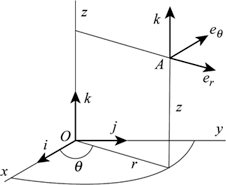# Coordinates 59763

The vectors v = (2.7; -1.8), w = (-3; 2.5) are given. Find the coordinates of the vectors: a = v + w, b = v-w, c = w-v, d = 2 / 3v

ax =  -0.3
ay =  0.7
bx =  5.7
by =  -4.3
cx =  -5.7
cy =  4.3
dx =  1.8
dy =  -1.2

### Step-by-step explanation:Did you find an error or inaccuracy? Feel free to write us. Thank you!

Tips for related online calculators
Our vector sum calculator can add two vectors given by their magnitudes and by included angle.